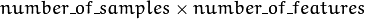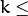# ml.Machine Learning¶

## ocl::KNearestNeighbour¶

class ocl::KNearestNeighbour : public ocl::CvKNearest

The class implements K-Nearest Neighbors model as described in the beginning of this section.

## ocl::KNearestNeighbour¶

Computes the weighted sum of two arrays.

class CV_EXPORTS KNearestNeighbour: public CvKNearest
{
public:
KNearestNeighbour();
~KNearestNeighbour();

bool train(const Mat& trainData, Mat& labels, Mat& sampleIdx = Mat().setTo(Scalar::all(0)),
bool isRegression = false, int max_k = 32, bool updateBase = false);

void clear();

void find_nearest(const oclMat& samples, int k, oclMat& lables);

private:
/* hidden */
};


## ocl::KNearestNeighbour::train¶

Trains the model.

C++: bool ocl::KNearestNeighbour::train(const Mat& trainData, Mat& labels, Mat& sampleIdx=Mat().setTo(Scalar::all(0)), bool isRegression=false, int max_k=32, bool updateBase=false)
Parameters: isRegression – Type of the problem: true for regression and false for classification. maxK – Number of maximum neighbors that may be passed to the method CvKNearest::find_nearest(). updateBase – Specifies whether the model is trained from scratch (update_base=false), or it is updated using the new training data (update_base=true). In the latter case, the parameter maxK must not be larger than the original value.

The method trains the K-Nearest model. It follows the conventions of the generic CvStatModel::train() approach with the following limitations:

• Only CV_ROW_SAMPLE data layout is supported.
• Input variables are all ordered.
• Output variables can be either categorical ( is_regression=false ) or ordered ( is_regression=true ).
• Variable subsets (var_idx) and missing measurements are not supported.

## ocl::KNearestNeighbour::find_nearest¶

Finds the neighbors and predicts responses for input vectors.

C++: void ocl::KNearestNeighbour::find_nearest(const oclMat& samples, int k, oclMat& lables)
Parameters: samples – Input samples stored by rows. It is a single-precision floating-point matrix ofsize. k – Number of used nearest neighbors. It must satisfy constraint:CvKNearest::get_max_k(). labels – Vector with results of prediction (regression or classification) for each input sample. It is a single-precision floating-point vector with number_of_samples elements.

## ocl::kmeans¶

Finds centers of clusters and groups input samples around the clusters.

C++: double ocl::kmeans(const oclMat& src, int K, oclMat& bestLabels, TermCriteria criteria, int attemps, int flags, oclMat& centers)
Parameters: src – Floating-point matrix of input samples, one row per sample. K – Number of clusters to split the set by. bestLabels – Input/output integer array that stores the cluster indices for every sample. criteria – The algorithm termination criteria, that is, the maximum number of iterations and/or the desired accuracy. The accuracy is specified as criteria.epsilon. As soon as each of the cluster centers moves by less than criteria.epsilon on some iteration, the algorithm stops. attempts – Flag to specify the number of times the algorithm is executed using different initial labellings. The algorithm returns the labels that yield the best compactness (see the last function parameter). flags – Flag that can take the following values: KMEANS_RANDOM_CENTERS Select random initial centers in each attempt. KMEANS_PP_CENTERS Use kmeans++ center initialization by Arthur and Vassilvitskii [Arthur2007]. KMEANS_USE_INITIAL_LABELS During the first (and possibly the only) attempt, use the user-supplied labels instead of computing them from the initial centers. For the second and further attempts, use the random or semi-random centers. Use one of KMEANS_*_CENTERS flag to specify the exact method. centers – Output matrix of the cluster centers, one row per each cluster center.

## ocl::distanceToCenters¶

For each samples in source, find its closest neighour in centers.

C++: void ocl::distanceToCenters(const oclMat& src, const oclMat& centers, Mat& dists, Mat& labels, int distType=NORM_L2SQR)
Parameters: src – Floating-point matrix of input samples. One row per sample. centers – Floating-point matrix of center candidates. One row per center. distType – Distance metric to calculate distances. Supports NORM_L1 and NORM_L2SQR. dists – The output distances calculated from each sample to the best matched center. labels – The output index of best matched center for each row of sample.

The method is a utility function which maybe used for multiple clustering algorithms such as K-means.

Image Processing

Object Detection